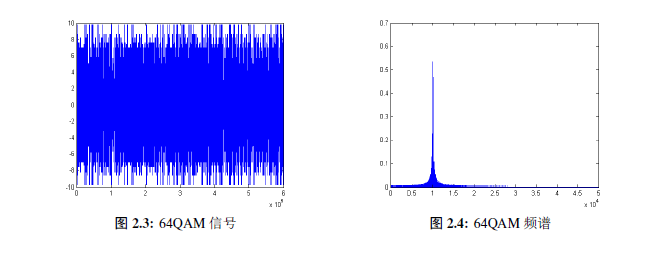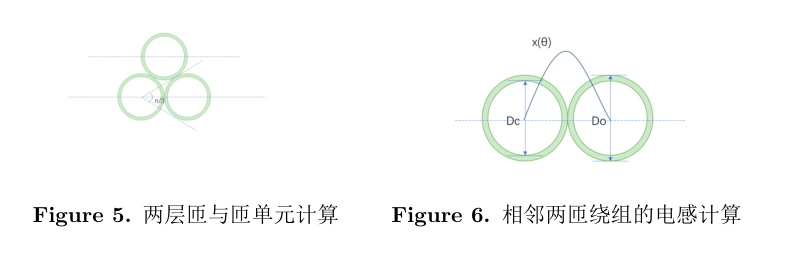• \begin{figure}[H] \begin{minipage}[t]{0.5\textwidth} \centering \includegraphics[scale=0.5]{1.png} ...H是在当前位置添加图片，需要在导言区调用float宏包：\usepackage{float} 效果

\begin{figure}[H]
\begin{minipage}[t]{0.5\textwidth}
\centering
\includegraphics[scale=0.5]{1.png}
\caption{64QAM信号\label{fig:1}}
\end{minipage}
\begin{minipage}[t]{0.5\textwidth}
\centering
\includegraphics[scale=0.5]{2.png}
\caption{64QAM频谱\label{fig:2}}
\end{minipage}
\end{figure}

H是在当前位置添加图片，需要在导言区调用float宏包：\usepackage{float}

效果图：展开全文• Latex 并排插入两张图片

千次阅读 2019-12-30 20:34:54
\begin{figure}[htbp] \centering \begin{minipage}[t]{0.48\textwidth} \centering \includegraphics[width=6cm]{Pic/pic_22} ...\caption{层匝与匝单元计算} \end{minipage} \begin{minipage}[t]{0.48\textw...
\begin{figure}[htbp]
\centering
\begin{minipage}[t]{0.48\textwidth}
\centering
\includegraphics[width=6cm]{Pic/pic_22}
\label{fig_22}
\caption{两层匝与匝单元计算}
\end{minipage}
\begin{minipage}[t]{0.48\textwidth}
\centering
\includegraphics[width=6cm]{Pic/pic_23}
\caption{相邻两匝绕组的电感计算}
\label{fig_23}
\end{minipage}
\end{figure}展开全文latex
• 图片并排显示 \begin{figure}[H] \centering \begin{minipage}{.5\textwidth} \centering \includegraphics[width=.8\linewidth]{images/logo1.png} \caption{Figure \#1} \label{fig:fig1} \end{minipage}%...

两张图片并排显示

\begin{figure}[H]
\centering
\begin{minipage}{.5\textwidth}
\centering
\includegraphics[width=.8\linewidth]{images/logo1.png}
\caption{Figure \#1}
\label{fig:fig1}
\end{minipage}%
\begin{minipage}{.5\textwidth}
\centering
\includegraphics[width=.8\linewidth]{images/logo2.png}
\caption{Figure \#2}
\label{fig:fig2}
\end{minipage}
\end{figure}

两张图片纵列显示，页码展示在左侧

\begin{figure}[H]
\centering
\includegraphics[scale=0.4]{images/chart.png}
\caption{Original Timeline}
\label{fig:chart}
\end{figure}

\begin{figure}[H]
\centering
\includegraphics[scale=0.4]{images/chart1.png}
\caption{Updated Timeline}
\label{fig:new_chart}
\end{figure}

展开全文latex
• latex并排显示多个图片

千次阅读 2020-11-24 22:16:27
下面以一行共同显示2个图片为例（可类推到多个图片）。 2.情况一 该情况共用一标题，有种方法： % 一行2个图片，共用1Figure标题 %方法1（较好），参考：...

1. 背景

并排图片分为两种情况，情况一是各子图共用一个标题（caption），该标题可以自己指定，每个子图的标题为a、b、c等，子图标题是系统生成的。情况二是各子图不共用标题，可设置不同的标题。下面以一行共同显示2个图片为例（可类推到多个图片）。

2.情况一

该情况共用一个标题，有两种方法：

% 一行2个图片，共用1个Figure标题
%方法1（较好），参考：https://blog.csdn.net/a6822342/article/details/80533135的第2段代码
\begin{figure}[htbp]
\centering

\subfigure[pic1.]{
\begin{minipage}[t]{0.45\linewidth} %linewidth小于0.5
\centering
\includegraphics[scale=0.4]{pdf/2.jpg}
%\caption{fig1}
\end{minipage}%
}%
\subfigure[pic2.]{
\begin{minipage}[t]{0.45\linewidth} %linewidth小于0.5
\centering
\includegraphics[scale=0.4]{pdf/2.jpg}
%\caption{fig2}
\end{minipage}%
}%

\centering
\caption{ pics}
\end{figure}
% 一行2个图片

% 一行2个图片，共用1个Figure标题
%方法2，参考：https://blog.csdn.net/a6822342/article/details/80533135的第3段代码，或者：https://zhuanlan.zhihu.com/p/32925549
\begin{figure}[H]
\centering  %图片全局居中
\subfigure[name1]{
\label{Fig.sub.1}
\includegraphics[scale=0.4]{pdf/2.jpg}}
\subfigure[name2]{
\label{Fig.sub.2}
\includegraphics[scale=0.4]{pdf/2.jpg}}
\caption{Main name}
\label{Fig.main}
\end{figure}
% 一行2个图片

3. 情况二

该情况不共用标题：

% 一行2个图片，不共用1个Figure标题，参考：https://www.zhihu.com/question/41322252的第一个(PPluvcoder)回答
\begin{figure}[htbp]
\centering
\begin{minipage}[t]{0.24\textwidth} %textwidth值小于0.25，或者linewidth小于0.5，不过这里设置textwidth比设置linewidth效果好一些
\centering
\includegraphics[scale=0.4]{pdf/2.jpg}
\caption{World Map}
\end{minipage}
\begin{minipage}[t]{0.24\textwidth}%textwidth值小于0.25
\centering
\includegraphics[scale=0.4]{pdf/2.jpg}
\caption{Concrete and Constructions}
\end{minipage}
\end{figure}

4. 控制图表大小

修改图片大小可以用widthscale命令，我习惯用scale命令。

%-----用width控制图片大小---------------
\begin{figure}[t]
\centering
\includegraphics[width = 0.2\linewidth]{pdf/1.png}
\caption{NER1}
\label{fig:NER_model1}
\end{figure}
%--------------figure end--------------

%-----用scale控制图片大小---------------
\begin{figure}[t]
\centering
\includegraphics[scale=0.2]{pdf/2.jpg}
\caption{NER2}
\label{fig:NER_model2}
\end{figure}
%--------------figure end--------------

6. 结尾

• 欢迎在评论区交流或者和我私信
• 如果您觉得我的文章对您有一些帮助，您可以请我喝一瓶饮料：展开全文latex
• Latex两张图片并列显示

千次阅读 2018-12-29 15:01:46
Latex的编辑中经常会遇到使得两张图片并列显示的情况。 %\usepackage{caption} %\usepackage{subfigure} %导言区域要添加以上两个包 \begin{figure}[htbp] \centering \subfigure[] { \begin{minipage...Latex
• LaTeX两张图并排显示

千次阅读 2020-04-08 17:42:14
\usepackage{caption} % 图片脚注 \usepackage{graphicx} \usepackage{caption} \usepackage{subfigure} % 子图包 二、具体代码 \begin{figure}[htbp] \subfigure[DogLeg Method] %第一张子 { \begin{...latex
• LaTeX一行排两个图片示例

千次阅读 2019-09-17 21:28:22
原博链接https://blog.csdn.net/manjhOK/article/details/83817907
•latex
• 这里写目录标题）正常插入图片并列插入图片 正常插入图片 \begin{figure} \includegraphics[width=0.5\textwidth]{figures/tree.eps} \caption{TGT_GTG​.} \label{tree} \end{figure} 如上述代码所示，首先要有...latex
• %图片1 \begin{tikzpicture}[scale=0.8]%用scale调整图片大小 \begin{axis}[ xlabel=x in $m^2$, ylabel=y in $m^2$, tick align=outside, y tick label style={/pgf/number format/.cd,% ...latex
• Latex 栏排版中图片、表格需要设置为一栏显示，在{figure}、{table}后加上 * \begin{figure*} …… \end{figure*} 实例如下： \begin{figure*}[!t] \centering \includegraphics[width=6in]{fig1.png} \label{Fig....latex
• latexdiff 可以对Latex文档中所作的修改进行高亮显示。 下面举栗子???? 首先我们有一origin.tex，内容如下： 接着我们又有一new.tex，内容如下： 我们很难看出两者的区别吧，对吧（请说：“对”），...程序人生 latex
• LaTeX如何让两张图并排显示

千次阅读 2020-03-28 15:47:49
转载 ...%\usepackage{caption} %\usepackage{subfigure} %导言区域要添加以上两个包 \begin{figure}[htbp] \centering %居中 \subfigure[name o...
• 如题, 分享一个latex中将图片分别作为一个图, 并将这张图并列放置的源代码. 其效果图如下图所示: 其源代码如下: \documentclass{article} \usepackage{graphicx} \begin{document} \begin{figure} \...
• LaTeX如何让两张图并排显示？

万次阅读 多人点赞 2018-01-30 15:14:22
%\usepackage{caption} ...%导言区域要添加以上两个包 \begin{figure}[htbp] \centering %居中 \subfigure[name of the subfigure] %第一张子 { \begin{minipage}{7cm} \centering %子图居中 \inLaTeX
• 本来想使用MathJax来生成LaTex公式，后来发现两个问题： MathJax太大； 生成的公式不是图片，无法存放在其它环境。 LaTex 本项目使用MathJax来渲染LaTex公式。 MathJax的删减 git拿下来的MathJax足足有152M。 根据...JavaScript
• LaTeX显示图像目录插入图片图片添加注解标签和引用 目录 插入图片 假设现在有张图片，路径为./images/universe.jpg，希望导入到文档中，代码如下。 \documentclass{article} \usepackage{graphicx} % 插入图片必要...latex
• 引入了宏包 \usepackage[caption=false,font=footnotesize,labelfont=sf,textfont=sf]{subfig} ...（1）两个图占一列，另外一个图单独一列 \begin{figure} \centering \begin{minipage}{.44\columnwidth} ...
• LaTeX figures side by side [duplicate] 介绍了两个图Figure 1 和Figure 2并排显示和两个子图subfigure的并排显示； Placing TikZ drawings in a minipage side by side 介绍了两个子图并排显示时，caption不对齐的...latex Figure
• latex 画图

2017-02-18 09:34:38
latex自带的工具tikzpicture画图，比matlab好看。latex plot
• beamer首页经过一周努力，人生第一LaTex的beamer所制作的slide完工。萌生用beamer制作slide的想法主要是听了吴喜之老师说的话，大意是“我们不能过分依赖商业软件，要多学习开源软件，我这张幻灯片就是用LaTex做...LaTeX不显示页码
• Sometimes you need to divide up a figure over multiple floats, for instance because the figure is too ...然后显示为： 本文转载自：https://en.wikibooks.org/wiki/LaTeX/Floats,_Figures_and_Captionslatex 分页显示
• latex 中一页面有很多图片，超出一页就显示不出来了：请问怎么让后续图片显示在一新页面中？图片显示代码如下每行显示三张：\begin{figure}\centering\includegraphics[width=1.8 in]{images/Fig5_Sample11a.jpg...latex 分页
• LaTeX插入文件需要导入宏包： \usepackage{graphicx} \usepackage{subfigure} 最初的代码： \begin{figure}[htb] \centering \includegraphics[width=7cm,height=5cm] {the stock return rate of seven ...
• [版权申明] 非商业目的注明出处可自由转载，转载请...示例五、横排显示所有图片 示例六：横排显示，超出第二排显示 示例七：并排显示2×2 示例八：插入单个图片，并设置空间位置 注意： 前言 Latex的基本设置.latex ieee论文
• latex eps图片不显示

千次阅读 2019-11-03 22:11:17
注正常显示 网上找了好久也没找到好的解决办法 ...在cmd里打开eps文件所在目录，输入下面命令可以转换为pdf，在latex代码中插入pdf图片就可以显示 repstopdf --outfile=hjorth.pdf hjorth.eps ...
• 感谢博主的启发，使用了该博主提供的代码，的确可以实现张表格并排，但是有问题就是，我加入了\caption{表格注释} 总是失败，排查了一下，发现原因是因为这位博主使用的代码块是\begin{minipage}，这代码块是...latex
• Latex中插入eps图片不显示，显示空白

万次阅读 多人点赞 2018-12-10 17:28:53
Latex中插入eps图片变异后，生成的pdf文件中，图片为空白 ...但是不要像下面这样，可能添加了上面代码中的两个包，依然显示空白，有可能是因为，两个包的顺序反了，如下，编译之后就可能得到空白的...latex...